# Math in Focus Grade 3 Cumulative Review Chapters 13 to 15 Answer Key

This handy Math in Focus Grade 3 Workbook Answer Key Cumulative Review Chapters 13 to 15 provides detailed solutions for the textbook questions.

## Math in Focus Grade 3 Cumulative Review Chapters 13 to 15 Answer Key

Concepts and Skills

Jessica is surveying and graphing her friends’ favorite foods.

Use the data in the tally chart to complete the bar graph. Then answer the question. (Lesson 13.1)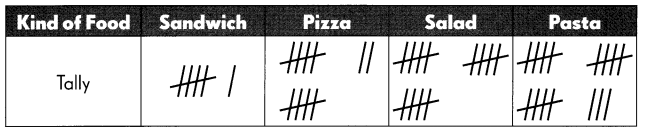Question 1.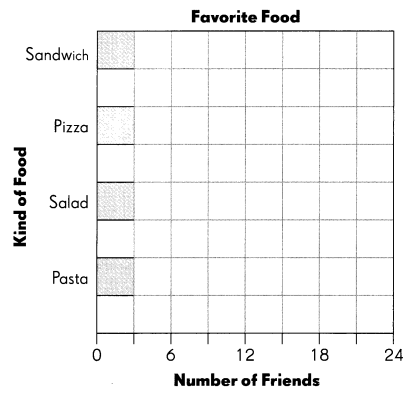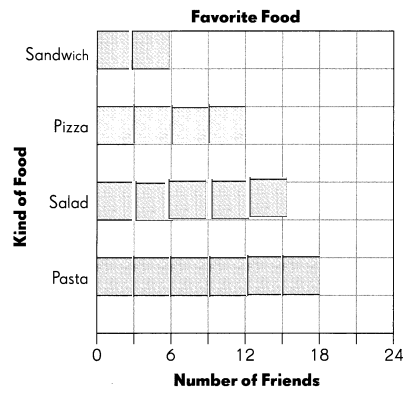Question 2.
How many friends did Jessica survey? ___________
6 + 12 + 15 + 18 = 51
Jessica surveyed 51 friends Favorite food.

Complete. (Lesson 14.1)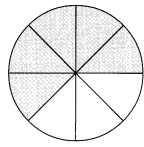Question 3.
Explanation:
In the above image we can observe 5 parts are shaded out of 8 parts. The shaded fraction is 5/8.

Question 4.
The numerator of the fraction is ___________.
The numerator of the fraction is 5.

Question 5.
The denominator of the fraction is ___________.
The denominator of the fraction is 8.

Write the missing numerator or denominator. (Lesson 14.2)

Question 6.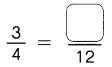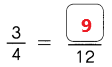Explanation:
In the above image we can observe the fraction 3/4. Multiply numerator and denominator with 3 the fraction is 9/12. The missing numerator is 9.

Question 7.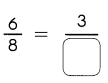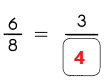Explanation:
The fraction 6/8 is equal to 3/4. The missing denominator is 4.

Question 8.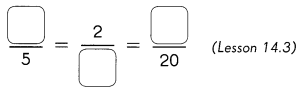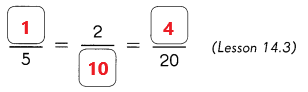Explanation:
Multiply the numerator and denominator with 2. So, the fractions are 1/5 = 2/10 = 4/20. The missing numerators are 1, 4. The missing denominator is 10.

Question 9.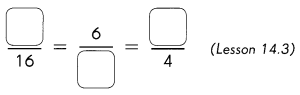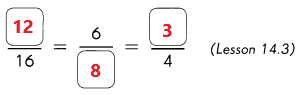Explanation:
In the above image we can observe some fractions. The fraction 12/16 is equal to 6/8 and 3/4. So, the missing numerators are 12, 6, 3. The missing denominator is 8.

Complete. Express each fraction in simplest form. (Lesson 14.3)

Question 10.
$$\frac{6}{10}$$ = ___________
6/10 = 3/5
Explanation:
The simplest form of the fraction 6/10 is 3/5.

Question 11.
$$\frac{8}{12}$$ = _____________
8/12 = 2/3
Explanation:
The simplest form of the fraction 8/12 is 2/3.

Circle the fraction that is greater. (Lesson 14.4)

Question 12.
$$\frac{5}{6}$$ or $$\frac{2}{3}$$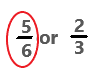Explanation:
The fraction 5/6 is greater than 2/3. So, draw a circle for 5/6.

Question 13.
$$\frac{2}{5}$$ or $$\frac{5}{8}$$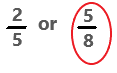Explanation:
The fraction 5/8 is greater than 2/5. So, draw a circle for 5/8.

Circle the fraction that is less. (Lesson 14.4)

Question 14.
$$\frac{5}{7}$$ or $$\frac{5}{9}$$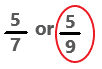Explanation:
The fraction 5/9 is less than 5/7. So, draw a circle for 5/9.

Question 15.
$$\frac{4}{9}$$ or $$\frac{5}{7}$$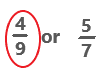Explanation:
The fraction 4/9 is less than 5/7. So, draw a circle for 4/9.

Compare. Write > or <. (Lesson 14.4)

Question 16.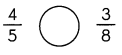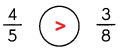Explanation:
The fraction 4/5 is greater than 3/8.

Question 17.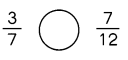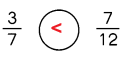Explanation:
The fraction 3/7 is less than 7/12.

Order the fractions from least to greatest. (Lesson 14.4)

Question 18.
$$\frac{1}{2}$$, $$\frac{3}{8}$$, $$\frac{7}{12}$$ _____________
The fractions from least to greatest are 3/8, 1/2, 7/12.

Order the fractions from greatest to least.

Question 19.
$$\frac{1}{6}$$, $$\frac{3}{4}$$, $$\frac{2}{3}$$ ______________
The fractions from greatest to least are 3/4, 2/3, 1/6.

Complete. Show each fraction on the number line. (Lesson 14.4)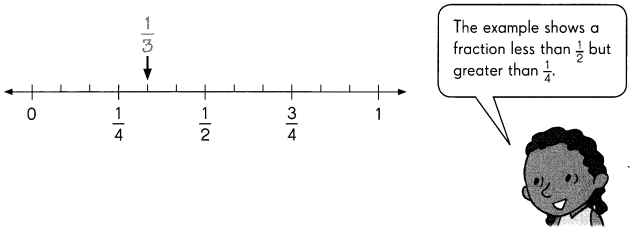Question 20.
A fraction greater than $$\frac{1}{2}$$ but less than $$\frac{3}{4}$$. ___________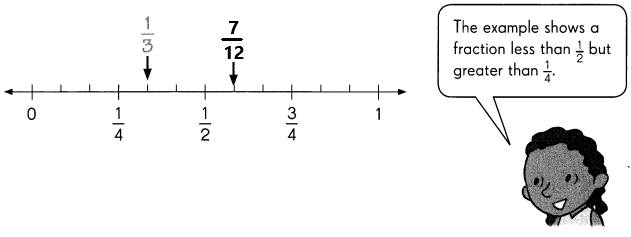1/2 + 1/12 = 7/12
A fraction greater than 1/2 but less than 3/4 is 7/12.

Question 21.
A fraction less than $$\frac{1}{6}$$ ___________.
A fraction less than1/6 is 1/7.

Question 22.
A fraction greater than $$\frac{5}{6}$$ _____________

Question 23.
$$\frac{1}{12}+\frac{5}{12}$$ = ____________
1/12 + 5/12 = 6/12 = 1/2

Question 24.
$$\frac{2}{6}+\frac{1}{6}+\frac{3}{6}$$ = _____________
2/6 + 1/6 + 3/6 = 6/6 = 1

Question 25.
$$\frac{5}{10}-\frac{4}{10}$$ = ____________
5/10 – 4/10 = 1/10

Question 26.
1 – $$\frac{5}{12}$$ = ____________
1 – 5/12 = (12 – 5)/12 = 7/12

Complete. Measure Line segment A to the nearest: (Lesson 15.1)

Question 27.
inch. _________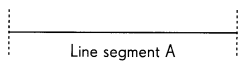Question 28.
half-inch. ____________

Color the pictures to find the fractional part of each set. (Lesson 14.6)

Question 29.
$$\frac{1}{2}$$ of 10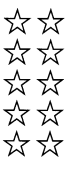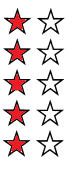Explanation:
1/2 of 10 = 5
Shaded 5 stars with out of 10.

Question 30.
$$\frac{3}{8}$$ of 16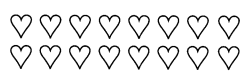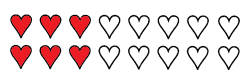Explanation:
3/8 of 16 = 6
Shaded 6 heart shape symbols out of 16.

Color the pictures to find the fractional part of each set. (Lesson 14.6)

Question 31.
$$\frac{1}{4}$$ of 20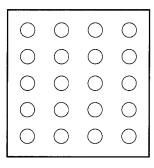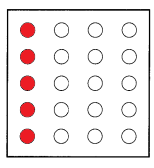Explanation:
1/4 of 20 = 5
In the above image we can observe 5 circles are shaded out of 20.

Question 32.
$$\frac{5}{6}$$ of 24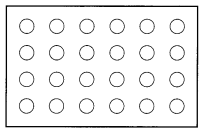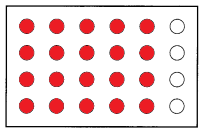Explanation:
5/6 of 24 = 20
In the above image we can observe 20 circles are shaded out of 24.

Write a fraction for the part of each set that is shaded. (Lesson 14.6)

Question 33.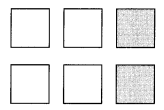In the above image we can observe 2/6 fraction part is shaded.

Question 34.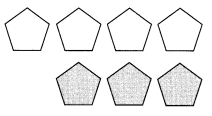In the above image we can observe 3/7 fraction part is shaded.

Choose the best unit of measure for measuring each length. Write inch, foot, yard, or mile. (Lesson 15.1)

Question 35.
width of a finger _______________
width of a finger inch.

Question 36.
length of a baseball bat _______________
length of a baseball bat inches.

Question 37.
length of a playground _______________
length of a playground foot.

Question 38.
distance walked in 1 hour _______________
distance walked in 1 hour Mile.

Complete. (Lesson 15.2)

Question 39.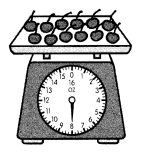The cherries weigh ___________ ounces.
The cherries weigh 8 ounces.
Explanation:
In the above image we can observe the pointer is at 8oz. So, the cherries weigh 8 ounces.

Question 40.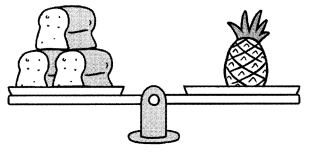The pineapple weighs about ___________ pounds.
The pineapple weighs about 3 pounds.
Explanation:
In the above image we can observe 3 loaf of bread in left side and pineapple in right side weighing balance. Each loaf of bread weighs about 1 pound. So, the pineapple weigh about 3 pounds.

Choose the best unit of measure for weighing each item. Write ounce, pound, or ton. (Lesson 15.2)

Question 41.
weight of a dog ___________
weight of a dog pounds.

Question 42.
weight of a box of toothpicks ___________
weight of a box of toothpicks pound.

Question 43.
weight of a hippopotamus ___________
weight of a hippopotamus tons.

Compare. Write > or <. (Lesson 15.2)

Question 44.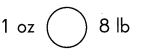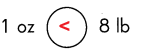Explanation:
1lb = 16oz
1 pound = 16ounce
So, 8lb is greater than 1oz.

Question 45.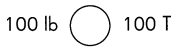Complete. (Lesson 15.3)

Question 46.
The pitcher is completely filled with water. The water is emptied into cups.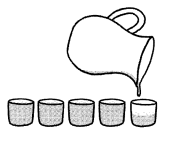The capacity of the pitcher is about ___________ cups.

Question 47.
5 pints of water are poured to fill a container.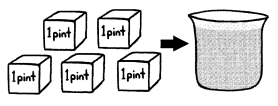The capacity of the container is about ___________ pints.
The capacity of the container is about 5  pints.

Circle the better estimate. (Lesson 15.3)

Question 48.
The capacity of a car’s fuel tank is 20 gallons / 20 pints.
The capacity of a car’s fuel tank is 8 pints.
Explanation:
We know that
1 gallon = 4 quarts
1 quart = 2 pints
First convert gallons into quarts.
20 gallons / 20 pints = (20 x 4) quarts / 20 pints
= 80 quarts / 20 pints
Convert quarts into pints.
= (80 x 2) pints / 20 pints
= 160 pints / 20 pints
= 8 pints

Question 49.
The amount of yogurt eaten for lunch is 1 cup / 1 quart.
The amount of yogurt eaten for lunch is 1/4 cups.
Explanation:
we know that
1 quart = 2 pints
1 pint = 2 cups
First convert quart into pints.
1 cup / 1 quart = 1 cup / (1 x 2) pints
= 1 cup / 2 pints
Next convert pints into cups.
1 cup / (2 x 2) cups = 1 cup / 4 cups
= 1/4 cups

Order from greatest capacity to least capacity. (Lesson 15.3)

Question 50.
6 pt 14 qt 2 gal
The order from the greatest capacity to least capacity is 14qt 2gal  6pt.
Explanation:
1 quart = 2 pints
1 gallon = 2 quarts
Convert quart into pints
14 quart = 14 x 2 pints = 28 pints
Convert gallons into pints
2 gallons = 2 x 4 x 2 pints = 16 pints
14qt > 2gal > 6pt

Question 51.
1 gal 18 c 10 pt
The order from the greatest capacity to least capacity is 10pt 18c 1gal.
Explanation:
1 pint = 2 cups
1 quart = 2 pints
1 gallon = 2 quarts
Convert gal into cups
1 gallon = 4 x 2 x 2 = 16 cups
Convert pints into cups
10 pints = 10 x 2 = 20 cups
10pt > 18C > 1gal

Problem Solving

Miguel conducted a survey to find the number of hours that some students spend playing their favorite sport each week.
He recorded the data in a table.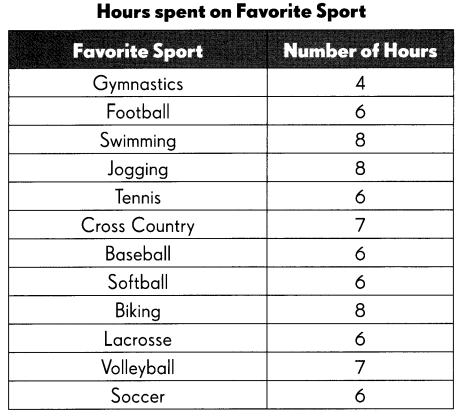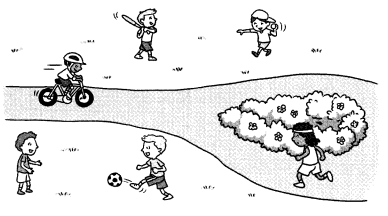Show the data in a line plot. Give your line plot a title.

Question 52.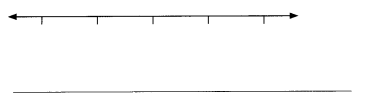Question 53.
How many students spend more than 5 hours on their favorite sport? ___________

Question 54.
How many hours did most students spend on their favorite sport? ___________ hours

Question 55.
The number of students who spent 6 hours on their favorite sport is ___________ times the number of students who spent 7 hours.

Question 56.
There are ___________ fewer students who spent 5 hours on their favorite sport than those who spent 8 hours.

Question 57.
If a total of 1 5 students were surveyed, how many students do not spend any time on sports? ___________

The bar graph shows the number of points scored by four basketball players.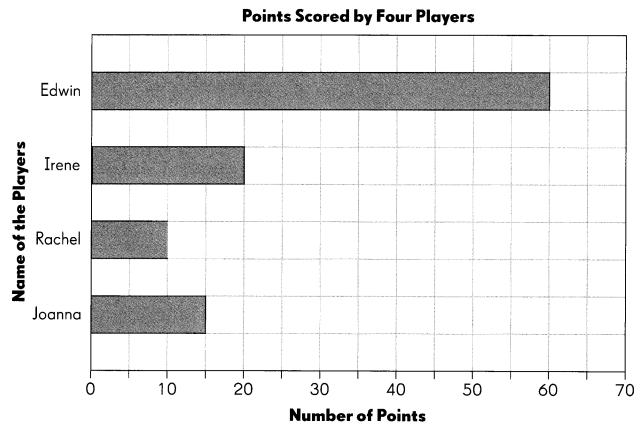Use the bar graph to answer the questions.

Question 58.
How many points did Edwin score?
Edwin scored 60 points.

Question 59.
Edwin scored 20 more points than Rachel. How many points did Rachel score?
Edwin scored 20 more points than Rachel. So, Rachel scored 40 points.

Question 60.
Use your answer from Exercise 59 to complete the bar graph for Rachel.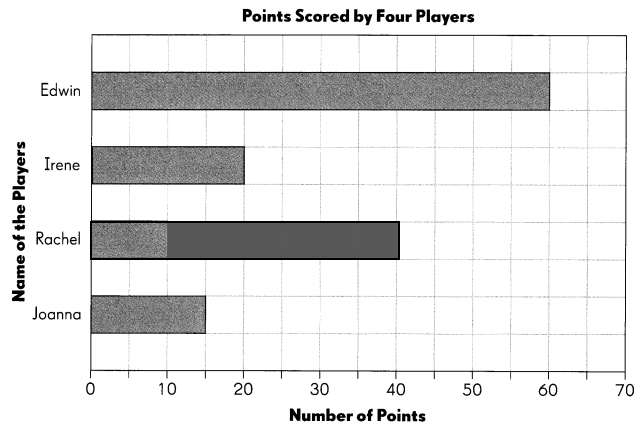Question 61.
Edwin scored three times as many points as one of the players. Who is this player?